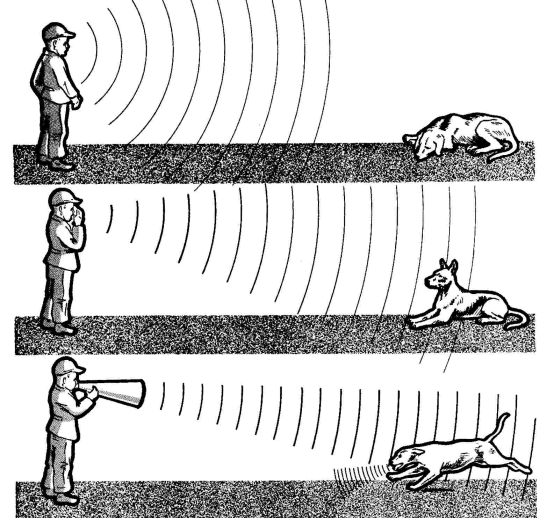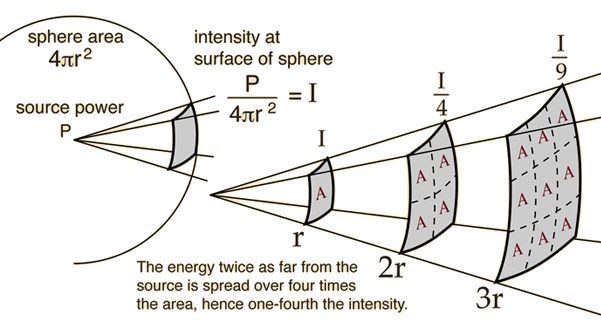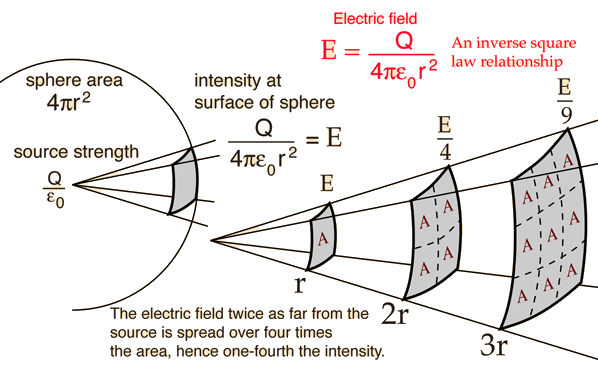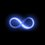# Inverse Square Law - A Visual ExplanationHas this ever bugged you? Are you sick of the lame old explanations with spray cans and flashlights? Does this law simply not make sense to you? You've come to the right place.

A picture's worth a thousand words. Look at the picture below.Makes sense?

No? Let me try. First, we assume that whatever we're dealing with originates from a point or approximately a point source. Second, we assume that thing distributes itself evenly in all directions, as with gravity fields, electromagnetic fields, ideal sound waves, light from a bulb, etc.

Now, the general idea is, as the distance from the source increases, the intensity of the projection decreases by the square of the distance. The square of the distance AND $4\pi$! Why? Simple: $4\pi r^2$ is the surface area of a sphere. And, an object with a point source whose field of effect is distributed about uniformly will form a sphere around itself. Hence, as the volume of effect expands, its area of effect divides itself evenly about the surface area of the resultant sphere.

One way to practically make sense of this is to consider an electric field. The electric field strength is represented by arrows. Now imagine an electron with four electric field lines evenly distributed about the point charge. If you look at a 2D model, you can see that at just about the surface of the electron, the field lines very close to each other. Now look at a distance much farther away; the lines are much more separated. This indicates a decreasing field intensity.

If you imagine this with a spray can, except the can itself is just a metal ball, then (in a vacuum) as it sprays, the total number of particles will uniformly distribute radially outward in all directions (if we maintain the analogy to electric field lines). Hence, at any given point, the number of particles there will be the total original amount divided by the surface area of the 'sphere' that exists at the radius of separation from the ball $\left( \frac{\text{Total}}{4\pi r^2}\right)$. So you would get sprayed four times less if you stand twice as far with respect to an arbitrary point, and nine times less if you stand three times farther from the same point.

Also note that since $4\pi$ is constant, when we compare one radius of separation to the other, it applies to both and hence cancels. But it is important to note that this quantity is present for any uniformly distributed field in 3D space, for without it we wouldn't be dividing by the total surface area to find the portion of the original field intensity at that point.

Now practically of course we don't have such 'point' sources. However, many sources behave approximately as such, especially considering that the more round an object is, the more it behaves as so. This is evident from light-bulbs, that emit light in all directions approximately perfectly uniformly. And sound, where air disturbances radiate in all directions. And SPRAY CANS, whose point-sources spray particles from almost a 'portion of the sphere', if you'd like to think of it that way.

In fact, if you have a spherical object, it does behave exactly as a point source. This requires a bit of knowledge from physics (or vector calculus, or great imagination), but that's a topic for another day :)

Here's that same image, for electric fields:Note by John M.
6 years, 2 months ago

This discussion board is a place to discuss our Daily Challenges and the math and science related to those challenges. Explanations are more than just a solution — they should explain the steps and thinking strategies that you used to obtain the solution. Comments should further the discussion of math and science.

When posting on Brilliant:

• Use the emojis to react to an explanation, whether you're congratulating a job well done , or just really confused .
• Ask specific questions about the challenge or the steps in somebody's explanation. Well-posed questions can add a lot to the discussion, but posting "I don't understand!" doesn't help anyone.
• Try to contribute something new to the discussion, whether it is an extension, generalization or other idea related to the challenge.
• Stay on topic — we're all here to learn more about math and science, not to hear about your favorite get-rich-quick scheme or current world events.

MarkdownAppears as
*italics* or _italics_ italics
**bold** or __bold__ bold
- bulleted- list
• bulleted
• list
1. numbered2. list
1. numbered
2. list
Note: you must add a full line of space before and after lists for them to show up correctly
paragraph 1paragraph 2

paragraph 1

paragraph 2

[example link](https://brilliant.org)example link
> This is a quote
This is a quote
    # I indented these lines
# 4 spaces, and now they show
# up as a code block.

print "hello world"
# I indented these lines
# 4 spaces, and now they show
# up as a code block.

print "hello world"
MathAppears as
Remember to wrap math in $$ ... $$ or $ ... $ to ensure proper formatting.
2 \times 3 $2 \times 3$
2^{34} $2^{34}$
a_{i-1} $a_{i-1}$
\frac{2}{3} $\frac{2}{3}$
\sqrt{2} $\sqrt{2}$
\sum_{i=1}^3 $\sum_{i=1}^3$
\sin \theta $\sin \theta$
\boxed{123} $\boxed{123}$

## Comments

Sort by:

Top Newest

What is the spraycan explanation?

- 6 years, 2 months ago

Log in to reply

Well they basically tell you that when you spray with a spray can it will obey the inverse square law because it will be four times less intense at twice the distance. But this never rang any bell to anyone as to why or how the law works - at all. And I'm not understating with the explanation above - that's basically all the spraycan demonstration is.

- 6 years, 2 months ago

Log in to reply

If you've read this far, here's another one:Can you now apply this idea to the opening picture? Think in reverse. Then you should truly understand what this law's all about.

- 6 years, 2 months ago

Log in to reply

consider an imaginary sphere around the person the net 'flux'of sound passing through the sphere as a whole remains the same in all three cases,the only difference in these cases is the difference in the flux distribution over this sphere as a whole.ie:-case1:the distribution is almost uniform,in case three the distribution of 'flux' is concentrated only on one portion of the sphere,but in turn diminishes the 'flux' through other parts....

- 3 years, 3 months ago

Log in to reply

Nicely explained.

- 6 years, 2 months ago

Log in to reply

Thanks! :D

- 6 years, 2 months ago

Log in to reply

Nicely explained but 2nd one not making sense.

- 6 years, 2 months ago

Log in to reply

Which one is the second one?

- 6 years, 2 months ago

Log in to reply

The one with a sphere with 3 hits and 9 hits.

- 6 years, 2 months ago

Log in to reply

Oh - the catch is, that outer square is of same area as the inner square. Hence, the intensity, or, field lines per that same area will be less by the square of the distance. Since the radius is doubled, intensity is... Wait... Yeah that's wrong. It's supposed to be four times less. It seems that whoever created this picture simply took the square root of the number of lines in the first square. Yup, you're right, it's a mess.

But if you notice, that illustration violates a condition for inverse square law - the field of interest must be uniformly distributed about the point source. Here, lines are unevenly spaced, and hence, the law does not apply.

- 6 years, 2 months ago

Log in to reply

Thanks.

- 6 years, 2 months ago

Log in to reply

Pachaaaa

- 6 years, 2 months ago

Log in to reply

×

Problem Loading...

Note Loading...

Set Loading...